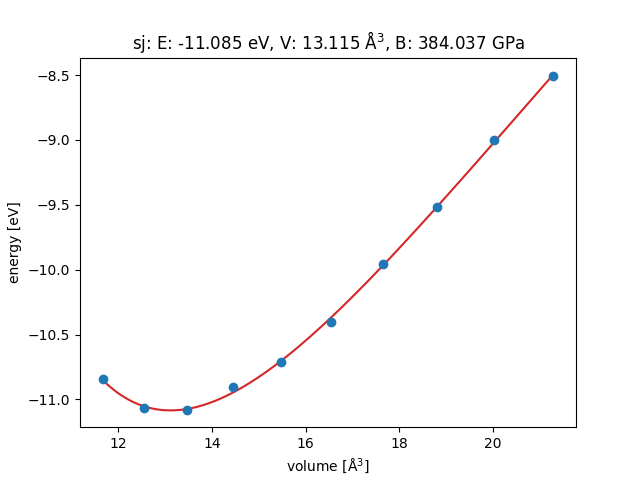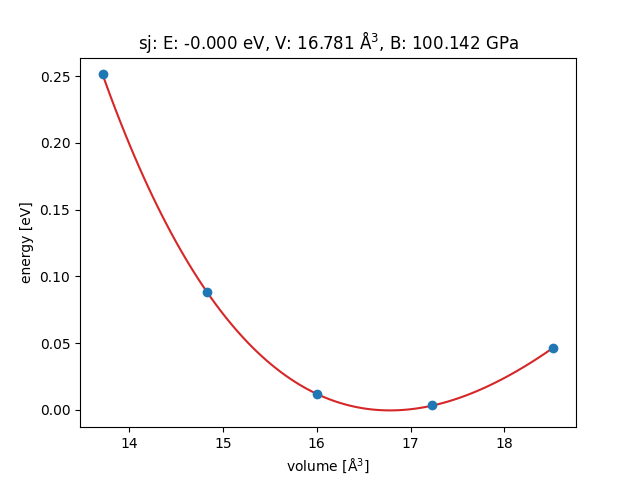# Lattice constant of Ag using GPAW

I followed the ASE tutorial for the equation of states of Ag from the official website of ASE, but tried to use the GPAW calculator for the potential energy of each configurations. Finally, I get the a bulk modulus value 384GPa, which is too large, and a lattice constant of 3.7 A, which is too small compared to the experimental value 4.08 A.
My code to calculate the potential energy in different lattice constant is here (with tiny change from the official example):

``````import numpy as np

from ase import Atoms
from ase.io.trajectory import Trajectory
from gpaw import GPAW

a = 4.0  # approximate lattice constant
b = a / 2
calc3 = GPAW(mode='pw', txt='gpaw.txt', xc='PBE')
ag3 = Atoms('Ag',
cell=[(0, b, b), (b, 0, b), (b, b, 0)],
pbc=1,
calculator=calc3)
cell = ag3.get_cell()
traj3 = Trajectory('Ag3.traj', 'w')
for x in np.linspace(0.9, 1.1, 10):
ag3.set_cell(cell * x, scale_atoms=True)
ag3.get_potential_energy()
print(ag3.get_potential_energy())
traj3.write(ag3)
``````

The fitting of energy vs. volume by EOS is like that:This is far from the result from the EMT potential:I tried to change the mode of GPAW, from ‘lcao’ to ‘pw’, but the result changed little.
I wonder what is wrong with that. My code is wrong or the GPAW got the wrong lattice constant and wrong bulk modulus?

You will need some k-points.

Best regards